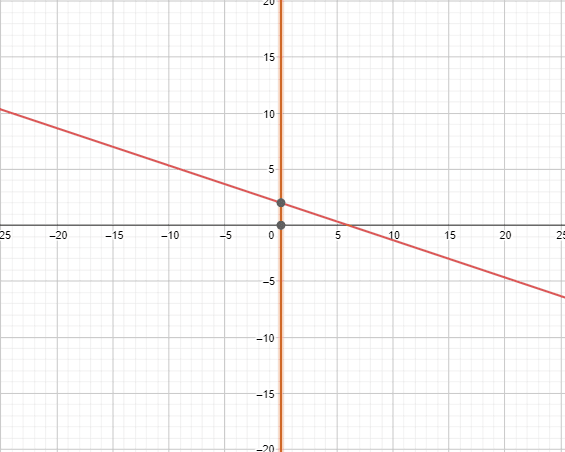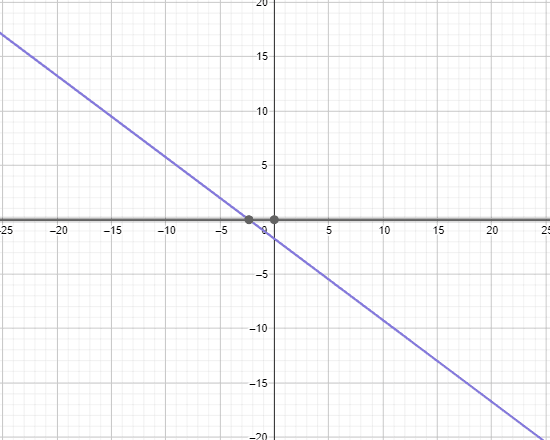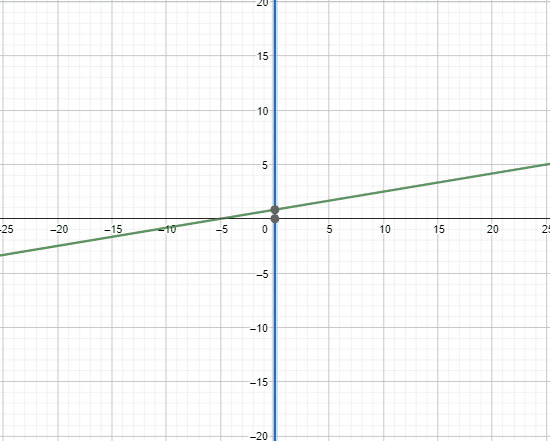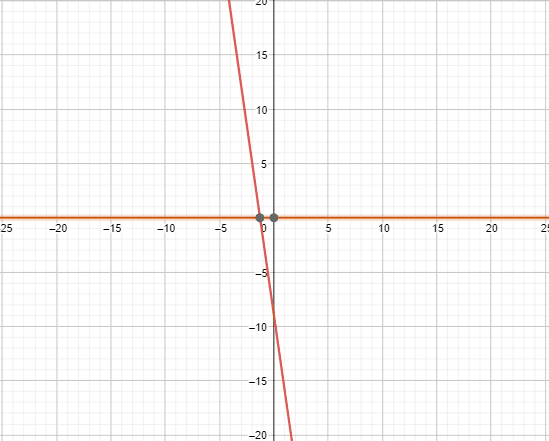Contents

• What Is an X and Y-intercept Calculator?
• How To Use the X and Y-intercept Calculator
• X and Y Intercepts
• How Does an X and Y Intercept Calculator Work?

Y-intercept Calculator + Online Solver With Free Steps

A y-intercept calculator is a calculator used to determine the point where a slope passes through the y-axis in an x-y plane.

Similarly, an x-intercept calculator finds out the point where a line crosses the x-axis. The calculator utilizes the equation y = mx + c to calculate the x or y-intercept.

The task of determining the intercepts manually is a tedious and lengthy process. It involves a lot of arithmetic operations and substitutions.

The x and y-intercept calculator makes this task easy as you only have to enter the equation into the calculator and select which intercept you want to calculate. The calculator provides a detailed solution as output. The output also displays a graph showing the intercepts in the x-y plane.

## What Is an X and Y-intercept Calculator?

An x and y-intercept Calculator is a helpful online tool used to determine the point on the x or y-axis where a straight line touches any of these axes.

It is highly useful as it can operate on any kind of equation entered into the calculator.

The calculator uses the internet to determine the intercepts. It reduces the lengthy process of solving the equation manually by merely entering the equation into the calculator. It makes the task of deciding the intercepts very easy.

The equation is entered into the calculator against the box titled Equation and the required intercept is entered in the space given against Find. On pressing the submit button, the step-by-step solution is displayed on the output window.

The x and y-intercept Calculator reduces the long process of finding intercepts into an operation of a few seconds.

## How To Use the X and Y-intercept Calculator

An x and y-intercept calculator is very efficient and easy to use. You can make use of this calculator by entering the desired equation and intercepts into the input boxes. The output screen displays the detailed solution as required by you.

The following steps are performed to obtain the x and y intercepts:

### Step 1

Determine an equation whose intercept needs to be determined. You need to keep in mind that the equation should be a line equation. That is, it should be in the form of y = mx + c.

### Step 2

An instruction is displayed on top of the calculator which says Enter the relationship as an equation with both x and y then select x-int or y-int. This instruction guides the user to enter an equation containing both variables x and y.

### Step 3

Enter the equation in the box titled Equation.

### Step 4

Two options are displayed against the title Find. You can scroll and select either y-intercept or x-intercept.

### Step 5

Press Submit to view the solution.

### Step 6

The output window displays the interpretation of the input in the form of equations written in the box against the title Intersection.

### Step 7

Below the heading Result, values of x and y are displayed. If the y-intercept is selected the value of x comes out to be 0 and if the x-intercept is chosen the value of y is 0.

### Step 8

The plot of the equation in the x-y plane is also displayed with the heading Implicit Plot. If the y-intercept is to be determined, the slope crosses a point on the y-axis and vice versa.

### Step 9

The step-by-step solution can also be viewed on the output screen.

### Step 10

The calculator can be used again and again to determine the intercepts by entering different equations.

## X and Y Intercepts

The concept of intercept in mathematics is that it is the point where a straight line or slope crosses the y-axis. A line is a geometric figure that exists in a two-dimensional space. Similarly, the x-axis and y-axis also exist in the x-y plane.

The y-intercept is the point where the line crosses the y-axis and the x-intercept is the point where the line crosses the x-axis. If one of the intercepts is kept zero, the other one can be determined.

## How Does an X and Y Intercept Calculator Work?

An x and y-intercept Calculator works by taking the equation containing both the intercepts as input into the calculator. By selecting among the options of x or y-intercept, the results can be easily obtained.

The calculator works by determining the actual points where the line or curve passes through the x or y-axis. This task can be performed manually by taking an equation with both the x and y variables in it. The equation is first converted into the line equation of the form y = mx + c. If the y-intercept is to be determined, the value of x is held to be zero. Similarly, if the x-intercept is to be determined, the value of y is substituted by zero.

The following process is adopted to find the intercepts manually:

The equation for a line is given in the form of:

ax + by + c = 0

The equation is solved for y. For this, the whole equation is divided by b.

\[ \dfrac{ax}{b} + \dfrac{by}{b} + \dfrac{c}{b}= \dfrac{0}{b} \]

\[ \dfrac{ax}{b} + y + \dfrac{c}{b} = 0 \]

\[ y = \dfrac{-ax}{b} + \dfrac{-c}{b} /]

This gives the equation for the y-intercept that is:

y = mx + c

Here,

\[ m = \dfrac{-a}{b} \] and \[ c = \dfrac{-c}{b} \]

Here,

m is the slope of the line and c is the y-intercept.

Now, to find the y-intercept let the value of x be 0, and to find the x-intercept take y as 0.

The x and y intercept calculator reduces this lengthy process to a few steps. The equation is entered and a detailed solution is obtained as output. The calculator provides results as follows:

### Input Interpretation

Under this heading, the calculator displays the entered equation where the line intersects the x and y axes.

### Result

The result displays the values of x and y on the screen. The result can be observed in approximate or accurate form. A step-by-step solution can also be obtained.

### Plot

The output window also displays the result in graphical form. The plot is developed in the x-y plane.

### Solved Examples

The following examples show how the x and y intercept calculator solves your problems efficiently:

### Example 1

Determine the y-intercept for the following equation:

2x + 6y = 12

### Solution

The y-intercept for the equation 2x + 6y = 12 is shown on the output screen as follows:

Intersections:

2x + 6y = 12

x = 0

#### Result

Substitute x = 0 into the equation 2x + 6y = 12.

6y = 12

\[ y = \dfrac{12}{6} \]

y = 2

The result is:

y = 2 and x = 0

#### Implicit PlotFigure 1

This shows that the y-intercept is y = 2

### Example 2

For the given equation:

-3x – 4y = 7

Find the x-intercept.

### Solution

The solution for the equation -3x – 4y = 7 is displayed as follows:

Intersections:

-3x – 4y = 7

y = 0

#### Result

By substituting y = 0 into the equation -3x – 4y = 7.

We get:

-3x = 7

\[ x = \dfrac{-7}{3} \]

The result is:

\[ x = \dfrac{-7}{3} \] and y = 0

#### Implicit PlotFigure 2

So, the x-intercept of the equation -3x – 4y = 7 is \[x = \dfrac{-7}{3} \]

### Example 3

Determine the y-intercept for the equation:

x – 6y = -5

### Solution

The y-intercept for the equation x – 6y = -5 is shown on the output screen as follows:

Intersections:

x – 6y = -5

x = 0

#### Result

Substitute x = 0 into the equation x – 6y = -5.

-6y = -5

\[ y = \dfrac{-5}{-6} /]

\[ y = \dfrac{5}{6} /]

The result is:

x = 0 and \[ y = \dfrac{5}{6} \]

#### Implicit PlotFigure 3

Hence, the y-intercept of the equation x – 6y = -5 is \[ y = \dfrac{5}{6}\]

### Example

Find the x-intercept of the line:

y = -7x – 9

### Solution

The x-intercept for the equation y = -7x – 9 is displayed as follows:

#### Input Interpretation

Following are some input interpretations.

y = -7x – 9

y = 0

#### Result

Substitute y = 0 into the equation y = -7x – 9.

-7x – 9 = 0

-7x = 9

\[ x = \dfrac{-9}{7} \]

The result is:

\[ x = \dfrac{-9}{7} \] and y = 0

#### Implicit PlotFigure 4

The x-intercept of the equation y = -7x – 9 is \[ x = \dfrac{-9}{7} \]

All the Mathematical drawings/images are created using GeoGebra.

You are watching: Y-intercept Calculator + Online Solver With Free Steps. Info created by GBee English Center selection and synthesis along with other related topics.